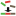# Normalization

This category contains 5 nodes.

##### Normalize Plates (B-Score)

The node performs a B-score normalization.

##### Normalize Plates (NPI)

The node performs a normalized-percent-of-inhibition(NPI)-normalization based on two subsets of the data (positive and negative control).

##### Normalize Plates (POC)

The node performs a percent-of-control(POC)-normalization based on a subset of the data.

##### Normalize Plates (Z-Score)

Performs a Z-score normalization based on a subset of the data.

##### Vector Length Normalization

Normalizes a vector to unit length. Vectors are defined by rows in the input table.